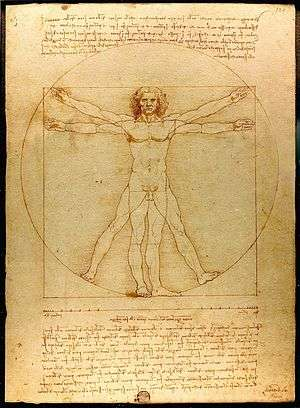# Scale (ratio)

The scale ratio of a model represents the proportional ratio of a linear dimension of the model to the same feature of the original. Examples include a 3-dimensional scale model of a building or the scale drawings of the elevations or plans of a building. In such cases the scale is dimensionless and exact throughout the model or drawing.

The scale can be expressed in four ways: in words (a lexical scale), as a ratio, as a fraction and as a graphical (bar) scale. Thus on an architect's drawing one might read

'one centimetre to one metre'    or    1:100   or    1/100

and a bar scale would also normally appear on the drawing.Da Vinci's Vitruvian Man illustrates the ratios of the dimensions of the human body; a human figure is often used to illustrate the scale of architectural or engineering drawings.

## General representation

In general a representation may involve more than one scale at the same time. For example, a drawing showing a new road in elevation might use different horizontal and vertical scales. An elevation of a bridge might be annotated with arrows with a length proportional to a force loading, as in 1 cm to 1000 newtons: this is an example of a dimensional scale. A weather map at some scale may be annotated with wind arrows at a dimensional scale of 1 cm to 20 mph.

## In maps

Main article: Map scale

Map scales require careful discussion. A town plan may be constructed as an exact scale drawing, but for larger areas a map projection is necessary and no projection can represent the Earth's surface at a uniform scale. In general the scale of a projection depends on position and direction. The variation of scale may be considerable in small scale maps which may cover the globe. In large scale maps of small areas the variation of scale may be insignificant for most purposes but it is always present. The scale of a map projection must be interpreted as a nominal scale. (The usage large and small in relation to map scales relates to their expressions as fractions. The fraction 1/10,000 used for a local map is much larger than 1/100,000,000 used for a global map. There is no fixed dividing line between small and large scales.)

A scale model is a representation or copy of an object that is larger or smaller than the actual size of the object being represented. Very often the scale model is smaller than the original and used as a guide to making the object in full size.
Unknown

## Mathematics

In mathematics, the idea of geometric scaling can be generalized. The scale between two mathematical objects need not be a fixed ratio but may vary in some systematic way; this is part of mathematical projection, which generally defines a point by point relationship between two mathematical objects. (Generally, these may be mathematical sets and may not represent geometric objects.)Wikimedia Commons has media related to Scales (ratio).